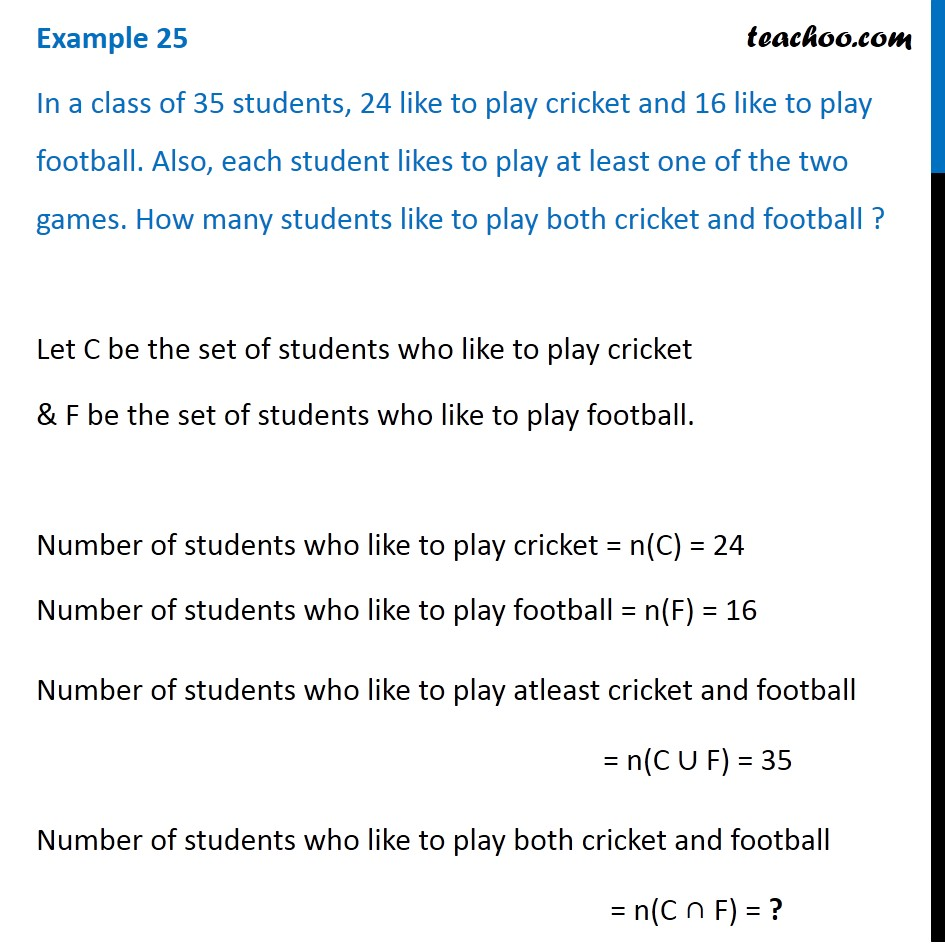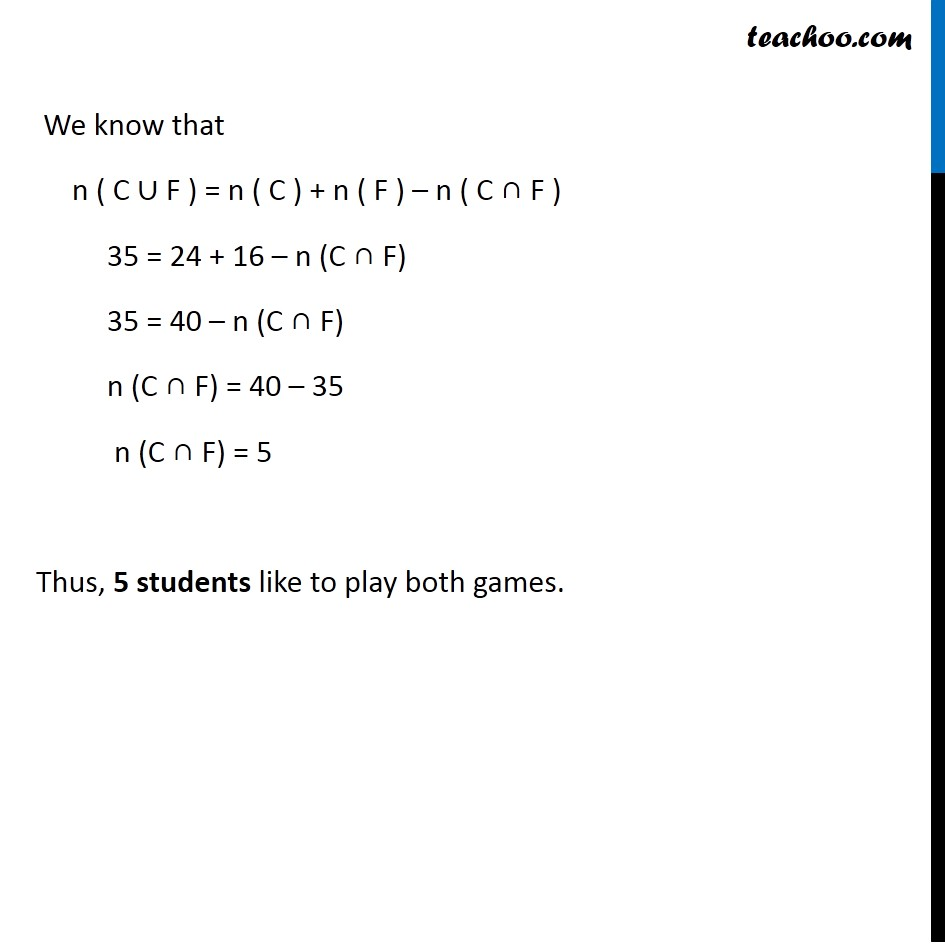1. Chapter 1 Class 11 Sets (Term 1)
2. Serial order wise
3. Examples

Transcript

Example 25 In a class of 35 students, 24 like to play cricket and 16 like to play football. Also, each student likes to play at least one of the two games. How many students like to play both cricket and football ? Let C be the set of students who like to play cricket & F be the set of students who like to play football. Number of students who like to play cricket = n(C) = 24 Number of students who like to play football = n(F) = 16 Number of students who like to play atleast cricket and football = n(C ∪ F) = 35 Number of students who like to play both cricket and football = n(C ∩ F) = ? We know that n ( C ∪ F ) = n ( C ) + n ( F ) – n ( C ∩ F ) 35 = 24 + 16 – n (C ∩ F) 35 = 40 – n (C ∩ F) n (C ∩ F) = 40 – 35 n (C ∩ F) = 5 Thus, 5 students like to play both games.

Examples

Chapter 1 Class 11 Sets (Term 1)
Serial order wise